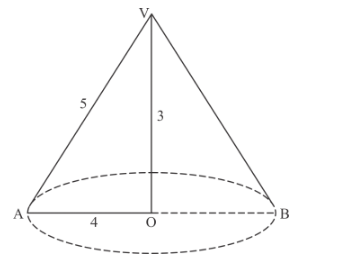# A right triangle with sides 3 cm,`
Question:

A right triangle with sides 3 cm, 4 cm and 5 cm is rotated about the side of 3 cm to form a cone. The volume of the cone so formed is

(a) 12π cm3

(b) 15π cm3

(c) 16π cm3

(d) 20π cm3

Solution:

r = 4 cm

Height of cone VAOB

h = 3 cmThe volume of cone VAOB

$=\frac{1}{3} \pi r^{2} h$

$=\frac{1}{3} \pi(3)^{2} \times 4$

$=12 \pi \mathrm{cm}^{3}$

Hence, the correct answer is choice (a).